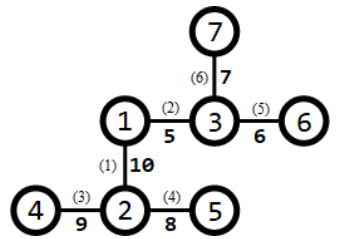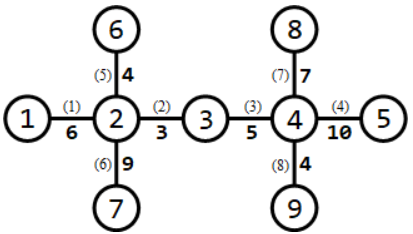# #NOIPS2018C. 赛道修建

ID: 79 Type: Default 1000ms 512MiB Tried: 14 Accepted: 6 Difficulty: 8 Uploaded By: Tags>NOIP 提高组2018

# 赛道修建

## 题目描述

C 城将要举办一系列的赛车比赛。在比赛前，需要在城内修建 $m$ 条赛道。

C 城一共有 $n$ 个路口，这些路口编号为 $1,2, \cdots , n$，有 $n − 1$ 条适合于修建赛道的双向通行的道路，每条道路连接着两个路口。其中，第 $i$ 条道路连接的两个路口编号为 $a_i$$b_i$，该道路的长度为 $l_i$。借助这 $n − 1$ 条道路，从任何一个路口出发都能到达其他所有的路口。

## 输出格式

7 1
1 2 10
1 3 5
2 4 9
2 5 8
3 6 6
3 7 7

31


## 样例说明 19 3
1 2 6
2 3 3
3 4 5
4 5 10
6 2 4
7 2 9
8 4 7
9 4 4

151. 经过第 $1,6$ 条道路的赛道（从路口 $1$ 到路口 $7$），长度为 $6 + 9 = 15$
2. 经过第 $5,2,3,8$ 条道路的赛道（从路口 $6$ 到路口 $9$），长度为 $4 + 3 + 5 + 4 = 16$
3. 经过第 $7,4$ 条道路的赛道（从路口 $8$ 到路口 $5$），长度为 $7 + 10 = 17$

## 数据范围与提示

$1$ $\le 5$ $=1$
$2$ $\le 10$ $\le n-1$
$3$ $\le 15$
$4$ $\le 10^3$ $=1$
$5$ $\le 3\times 10^4$
$6$
$7$ $\le n-1$
$8$ $\le 5\times 10^4$
$9$ $\le 10^3$
$10$ $\le 3\times 10^4$
$11$ $\le 5\times 10^4$
$12$ $\le 50$
$13$
$14$ $\le 200$
$15$
$16$ $\le 10^3$
$17$
$18$ $\le 3\times 10^4$
$19$
$20$ $\le 5\times 10^4$